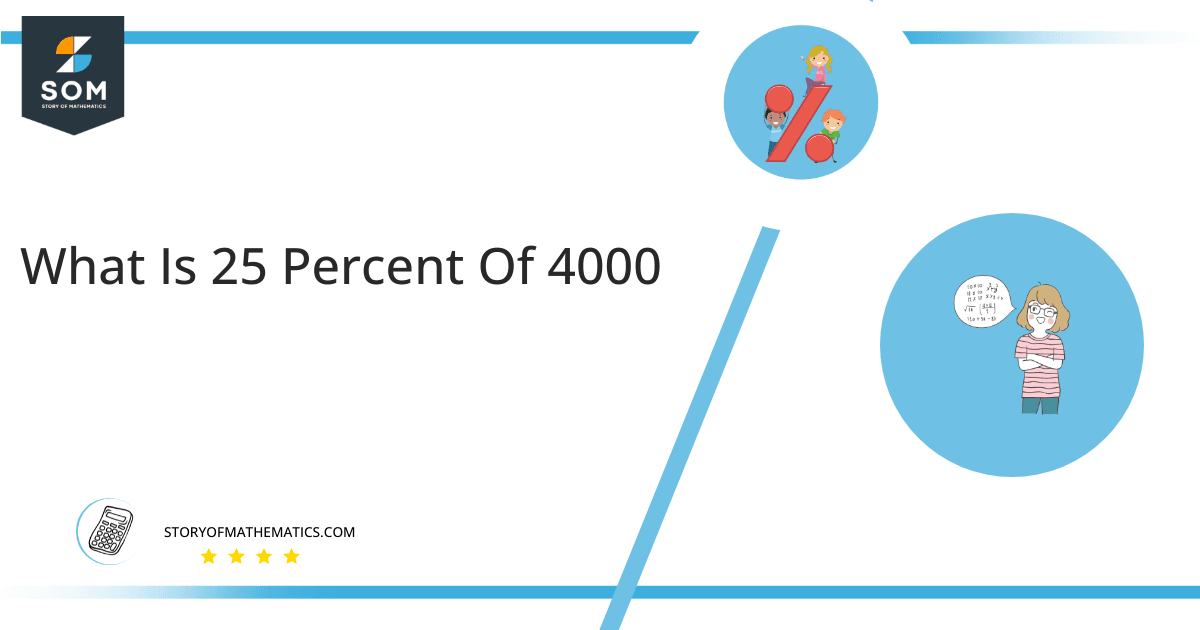# What Is 25 Percent of 4000 + Solution with Free Steps?

The 25 percent of 4000 is equal to 1000. It can be easily calculated by dividing 25 by 100 and multiplying the answer with 4000 to get 1000.The easiest way to get this answer is by solving a simple mathematical problem of percentages. You need to find 25% of 4000 for some sale or real-life problem. Divide 25 by 100, multiply the answer by 4000, and get the 25% of 4000 value in seconds.

This article will explain the full process of finding any percentage value from any given quantity or number with easy and simple steps.

## What Is 25 percent of 4000?

The 25 percent of 4000 is 1000.

The percentage can be understood with a simple explanation. Take 4000, and divide it into 100 equal parts. The 25 parts from the total of 100 parts is called 25 percent, which is 1000 in this example.

## How To Calculate 25 percent of 4000?

You can find 25 percent of 4000 by some simple mathematical steps explained below.### Step 1

Firstly, depict 25 percent of 4000 as a fractional multiple as shown below:

25% x 4000

### Step 2

The percentage sign % means percent, equivalent to the fraction of 1/100.

Substituting this value in the above formula:

= (25/100) x 4000

### Step 3

Using the algebraic simplification process, we can arithmetically manipulate the above equation as follows:

= (25 x 4000) / 100

= 100000 / 100

= 1000This percentage can be represented on a pie chart for visualization. Let us suppose that the whole pie chart represents the 4000 values. Now, we find 25 percent of 4000, which is 1000. The area occupied by the 1000 value will represent the 25 percent of the total 4000 values. The remaining region of the pie chart will represent 75 percent of the total 4000 values. The 100% of 4000 will cover the whole pie chart as 4000 is the total value.

Any given number or quantity can be represented in percentages to understand the total quantity better. The percentage can be considered a quantity that divides any number into hundred equal parts for better representation of large numbers and understanding.

Percentage scaling or normalization is a very simple and convenient method of representing numbers in relative terms. Such notations find wide application in many industrial sectors where the relative proportions are used.